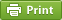﻿ Finding enthalpy change using Hess's Law

# Finding enthalpy change using Hess's LawChemists can determine the enthalpy change of any reaction using an important law, known as Hess’s law of heat summation. This law states that the enthalpy change of a physical or chemical process depends only on the beginning conditions (reactants) and the end conditions (products). The enthalpy change is independent of the pathway of the process and the number of intermediate steps in the process. It is the sum of the enthalpy changes of all the individual steps that make up the process.

Practice problem1:

Ethene, C2H4, reacts with water to form ethanol, CH3CH2OH(l) .
C2H4(g) + H2O(l) → CH3CH2OH(l)
Determine the enthalpy change of this reaction, given the following thermochemical equations.
(1) CH3CH2OH(l) + 3O2(g) → 3H2O(l) + 2CO2(g) ΔH° = −1367 kJ
(2) C2H4(g) + 3O2(g) → 2H2O(l) + 2CO2(g) ΔH° = −1411 kJ

Enthalpy change for the target equation can be determined by adding equation 1 and equation 2.

You need to flip the first equation so that CH3CH2OH goes to the product side. When ever you flip an equation the energy sign is revered.You dont need to flip the 2nd equation.

So, the 1st equation looks as follows after flipping.

3H2O(l) + 2CO2(g)→CH3CH2OH(l) + 3O2(g)    ΔH° = +1367 kJ

C2H4(g) + 3O2(g) → 2H2O(l) + 2CO2(g)             ΔH° = −1411 kJ

____________________________________________________

C2H4(g) + H2O(l) → CH3CH2OH(l)                         ΔH° =-44 kJ

___________________________________________________

Practice Problem 2:

A typical automobile engine uses a lead-acid battery. During discharge, the following chemical reaction takes place.
Pb(s) + PbO2(s) + 2H2SO4(l) → 2PbSO4(aq) + 2H2O(l)
Determine the enthalpy change of this reaction, given the following
equations.
(1) Pb(s) + PbO2(s) + 2SO3(g) → 2PbSO4(s) ΔH° = −775 kJ
(2) SO3(g) + H2O(l) → H2SO4(l)                         ΔH° = −133 kJ

1st equation don't need to be flipped . 2nd equation needs to be flipped and times by 2 to get 2 mol of H2SO4

Pb(s) + PbO2(s) + 2SO3(g) → 2PbSO4(s)                                ΔH° = −775 kJ

2 H2SO4(l)                                   →2SO3(g) + 2H2O(l)             ΔH° = +266 kJ

______________________________________________________

Pb(s) + PbO2(s) + 2H2SO4(l) → 2PbSO4(aq) + 2H2O(l)   ΔH° = -509 kJ

______________________________________________________

Practice Problem 3:

Mixing household cleansers can result in the production of hydrogen chloride gas, HCl(g). Not only is this gas dangerous in its own right, but it also reacts with oxygen to form chlorine gas and water vapour.
4HCl(g) + O2(g) → 2Cl2(g) + 2H2O(g)
Determine the enthalpy change of this reaction, given the following equations.
(1) H2(g) + Cl2(g) → 2HCl(g) ΔH° = −185 kJ
(2) H2(g) + 1/2 O2(g) → H2O(l) ΔH° = −285.8 kJ
(3) H2O(g) → H2O(l) ΔH° = −40.7 kJ

Note: A substance can be cancelled on both sides of the equation only when they both are in same phase(slid/liquid/gas)

Equation 1: Flip and times by 2.

Equation 2: Multiply by 2

Equation 3: Flip and multiply by 2

2HCl(g)→2H2(g) +2 Cl2(g)     ΔH° = +370 kJ

2H2(g) +  O2(g) →2 H2O(l)   ΔH° = −571.6kJ

2H2O(g) → 2H2O(l)                ΔH° = +81.4kJ

___________________________________________

4HCl(g) + O2(g) → 2Cl2(g) + 2H2O(g)    ΔH° = -120.2 kJ

___________________________________________

Practice problem 4:

Calculate the enthalpy change of the following reaction between nitrogen gas and oxygen gas, given thermochemical equations (1), (2), and (3).
2N2(g) + 5O2(g) → 2N2O5(g)

(1) 2H2(g) + O2(g) → 2H2O(l) ΔH° = −572 kJ
(2) N2O5(g) + H2O(l) → 2HNO3(l) ΔH° = −77 kJ
(3) 1/2 N2(g) + 3/2 O2(g) + 1/2 H2(g) → HNO3(l) ΔH° = −174 kJ

Equation 1: Leave it alone

Equation 2: flip and multiply by 2

Equation 3: Multiply by 4 ( you get 2 N2, 6 O2 on left side)

Equation 1: need to be flipped to cancel the oxygen.

2H2O(l)                                        → 2H2(g) + O2(g)            ΔH° = +572 kJ

4HNO3(l)                                  →2N2O5+2H2O(l)          ΔH° = +154 kJ

2 N2(g) + 6O2(g) + 2 H2(g)  → 4HNO3(l)                       ΔH° = −696 kJ

5O2

____________________________________________________

2N2(g) + 5O2(g) → 2N2O5(g)                                            ΔH° = +30kJ

____________________________________________________